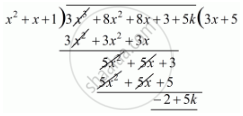Advertisement Remove all ads

# If X2 + X + 1 is a Factor of the Polynomial 3x3 + 8x2 + 8x + 3 + 5k, Then the Value of K is - Mathematics

MCQ

If x2 + x + 1 is a factor of the polynomial 3x3 + 8x2 + 8x + 3 + 5k, then the value of k is

#### Options

• 0

• 2/5

• 5/2

• -1

Advertisement Remove all ads

#### Solution

Let  f(x) = 3x^2 + 8x^2 + 8x+ 8x + 3 + 5k be the given polynomial,

Since  (x^2 + x + 1)is the factor of f(x). Therefore, remaider will be zero.

Now,Now,

-2 + 5k = 0

k=2/5`

Is there an error in this question or solution?
Advertisement Remove all ads

#### APPEARS IN

RD Sharma Mathematics for Class 9
Chapter 6 Factorisation of Polynomials
Q 17 | Page 35
Advertisement Remove all ads

#### Video TutorialsVIEW ALL 

Advertisement Remove all ads
Share
Notifications

View all notifications

Forgot password?
Course